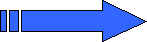X

PHPSimplex
Version 0.81

Developed by:
Daniel Izquierdo Granja
Juan José Ruiz Ruiz

English translation by:
Luciano Miguel Tobaria

French translation by:
Ester Rute Ruiz

Portuguese translation by:
Rosane Bujes

PHPSimplex

• Start
• Theory
• Example
• Help
• Exit

PHPSimplex

The problem is converted to canonical form by adding slack, surplus and artificial variables as appropiate (show/hide details)

• As the constraint 1 is of type '≥' we should add the surplus variable X3 and the artificial variable X7.
• As the constraint 2 is of type '≥' we should add the surplus variable X4 and the artificial variable X8.
• As the constraint 3 is of type '≥' we should add the surplus variable X5 and the artificial variable X9.
• As the constraint 4 is of type '≥' we should add the surplus variable X6 and the artificial variable X10.
 MINIMIZE: Z = 0.2 X1 + 0.08 X2MAXIMIZE: Z = -0.2 X1 -0.08 X2 + 0 X3 + 0 X4 + 0 X5 + 0 X6 + 0 X7 + 0 X8 + 0 X9 + 0 X10 subject to 0.1 X1 + 0 X2 ≥ 0.40 X1 + 0.1 X2 ≥ 0.60.1 X1 + 0.2 X2 ≥ 20.2 X1 + 0.1 X2 ≥ 1.7 subject to 0.1 X1 -1 X3 + 1 X7 = 0.40 X1 + 0.1 X2 -1 X4 + 1 X8 = 0.60.1 X1 + 0.2 X2 -1 X5 + 1 X9 = 20.2 X1 + 0.1 X2 -1 X6 + 1 X10 = 1.7 X1, X2 ≥ 0 X1, X2, X3, X4, X5, X6, X7, X8, X9, X10 ≥ 0

We'll build the first tableau of Phase I from Two Phase Simplex method.